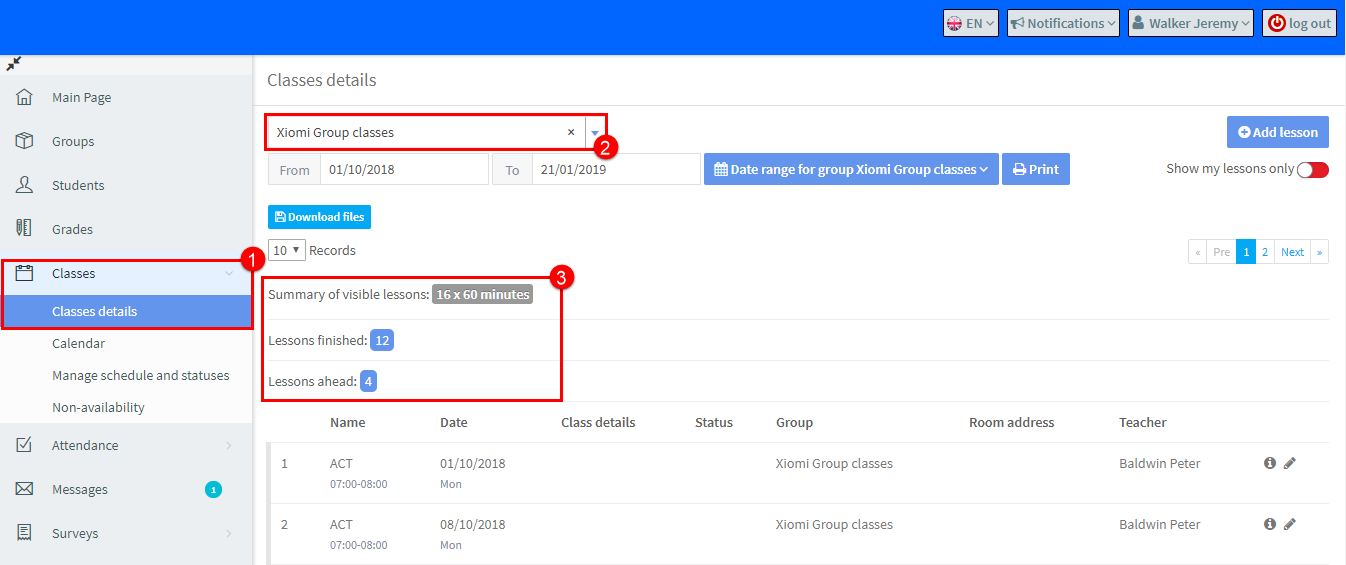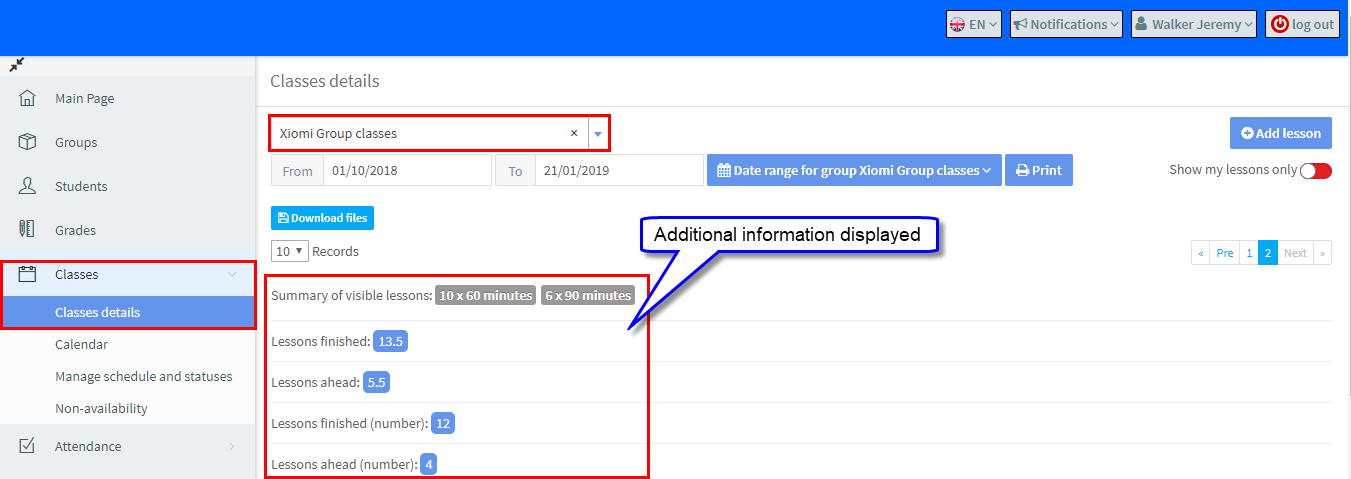# How many lessons are completed and how many remain until the end of the course?

January 4, 2019

To find out how many lessons have taken place and how many remain:

1. Go to Classes – Class Details.
2. Select the Group.
3. And the lessons finished and remaining are shown.“Lessons finished” is the sum of lessons from the beginning of the course to the current date, excluding the cancelled classes.

To calculate lesson amount, the system takes into account the default lesson length. If the duration of a class is different from the default lesson length, additional information about the current lesson number will be displayed.